# TExES Physics/Math 7-12: Geometry and Measurement Chapter Exam

Exam Instructions:

Choose your answers to the questions and click 'Next' to see the next set of questions. You can skip questions if you would like and come back to them later with the yellow "Go To First Skipped Question" button. When you have completed the practice exam, a green submit button will appear. Click it to see your results. Good luck!

Answered 0 of 20

### Page 1

#### Question 1 1. Given f(x) on the graph below, which of the following integrals will equal 0?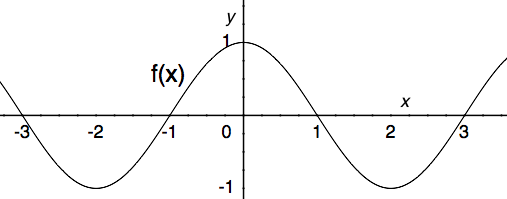#### Question 2 2. Which of the following represents the area of the first slice under the curve f(x) between x = 0 and x = 2 when divided into 4 slices?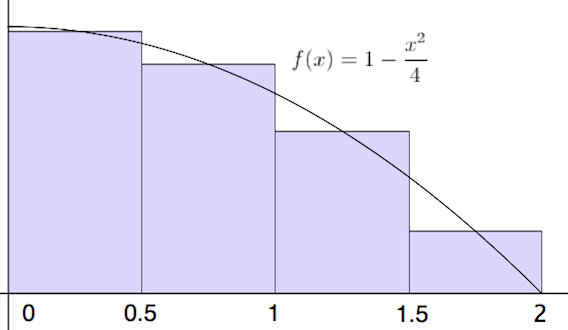#### Question 4 4. Which of the following graphs shows the correct region of integration for the integral below?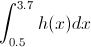#### Question 5 5. Estimate the area beneath the curve f(x) using the two term Riemann sum as shown below.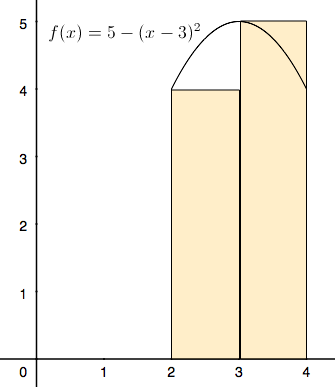### Page 2

#### Question 6 6. You are going to take a Riemann Sum of the area below. To do this, you divide the region into four slices of equal width. How wide is each slice?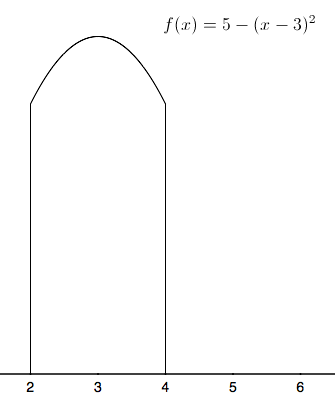#### Question 7 7. Write out the first four terms of a Riemann sum equivalent to the following integral.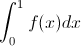#### Question 8 8. How many terms are in the Riemann sum represented below?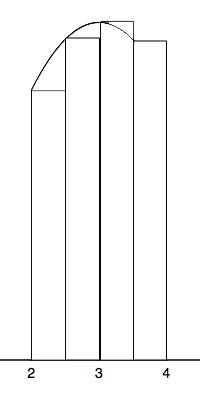#### Question 9 9. In the following integral, 4 is defined as which of the following?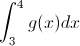#### Question 10 10. Which of the following integrals represents the area under the graph below?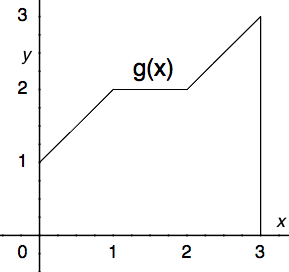### Page 3

#### Question 11 11. Given f(x) on the graph, what does z equal?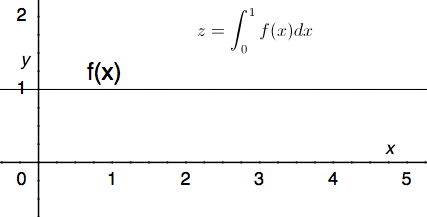#### Question 12 12. Which integral below is equal to the following integral?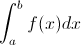#### Question 13 13. In the following integral, g(x) is defined as which of the following?#### Question 14 14. Given the following information, what does z equal?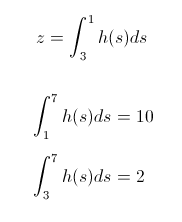#### Question 15 15. Using the single term Riemann sum as shown, what is the area beneath the curve f(x) between 0 and two pi?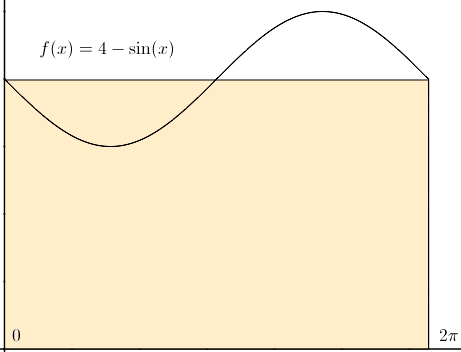### Page 4

#### Question 16 16. Which integral below is equal to the following integral?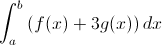#### Question 17 17. Which of the following integrals is equal to the following summation over the continuous region x=a to x=b?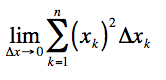#### Question 18 18. The following integral can be re-written as: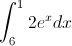#### Question 19 19. Which of the following integrals is equal to the following summation over the continuous region x=a to x=b?#### Question 20 20. Evaluate the following.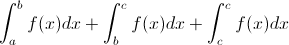#### TExES Physics/Math 7-12: Geometry and Measurement Chapter Exam Instructions

Choose your answers to the questions and click 'Next' to see the next set of questions. You can skip questions if you would like and come back to them later with the yellow "Go To First Skipped Question" button. When you have completed the practice exam, a green submit button will appear. Click it to see your results. Good luck!

Support